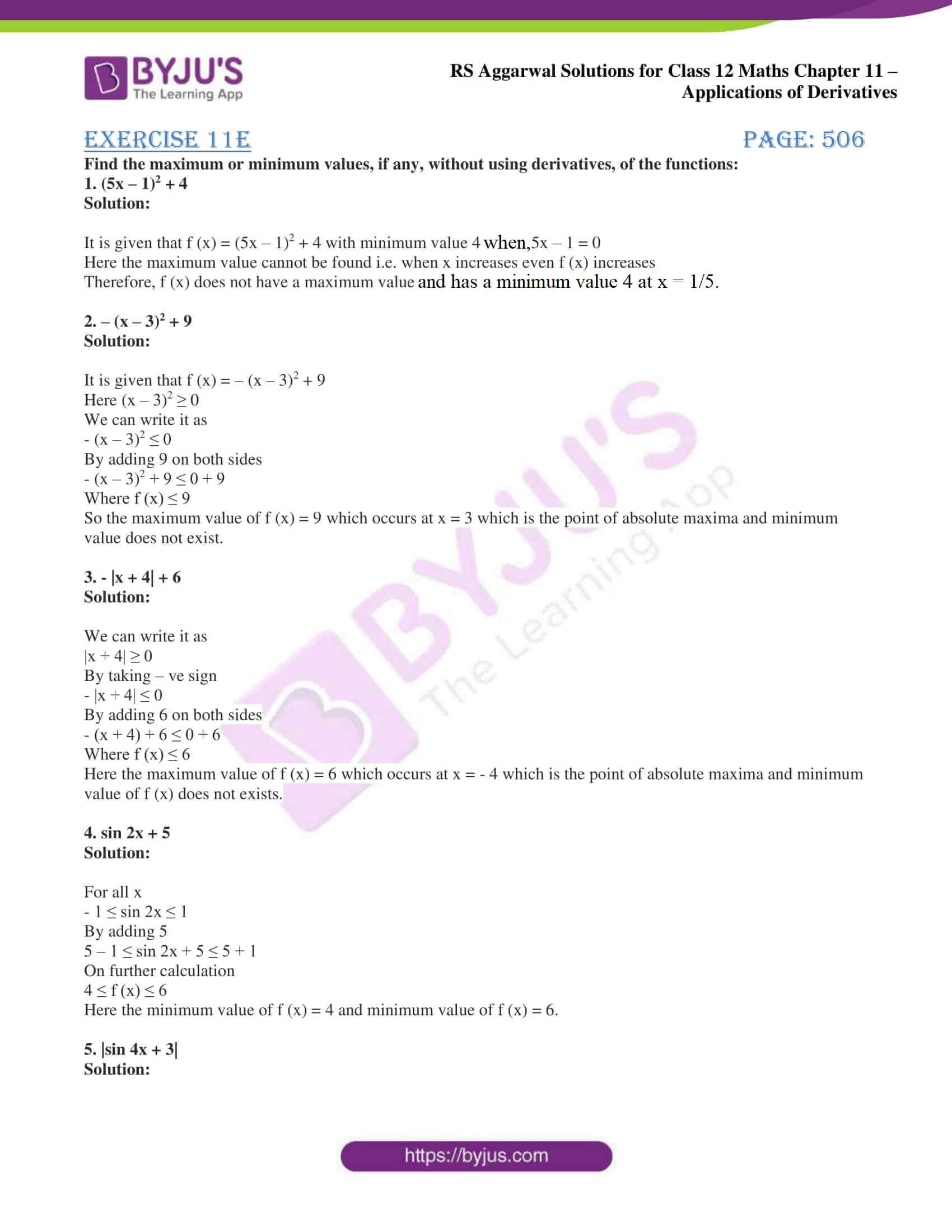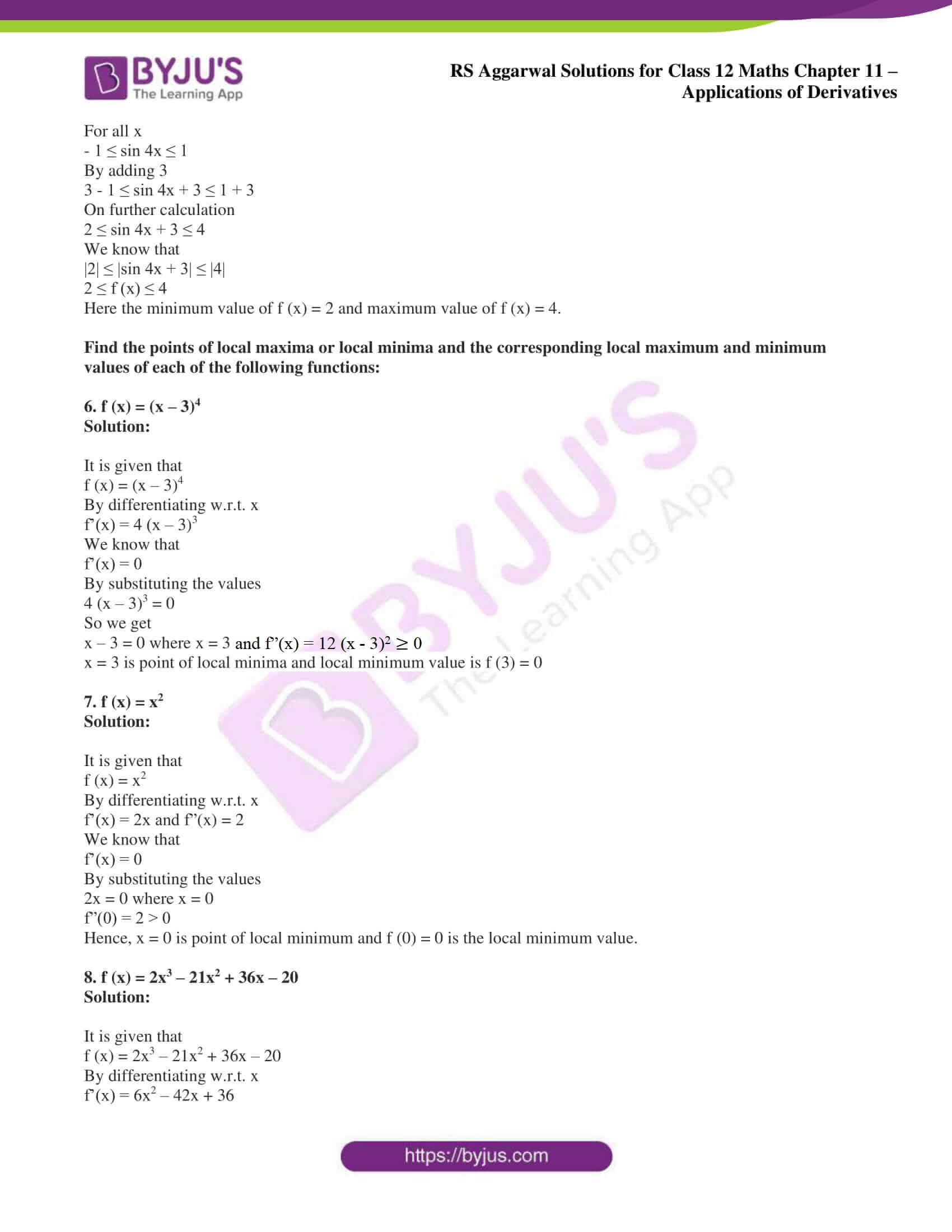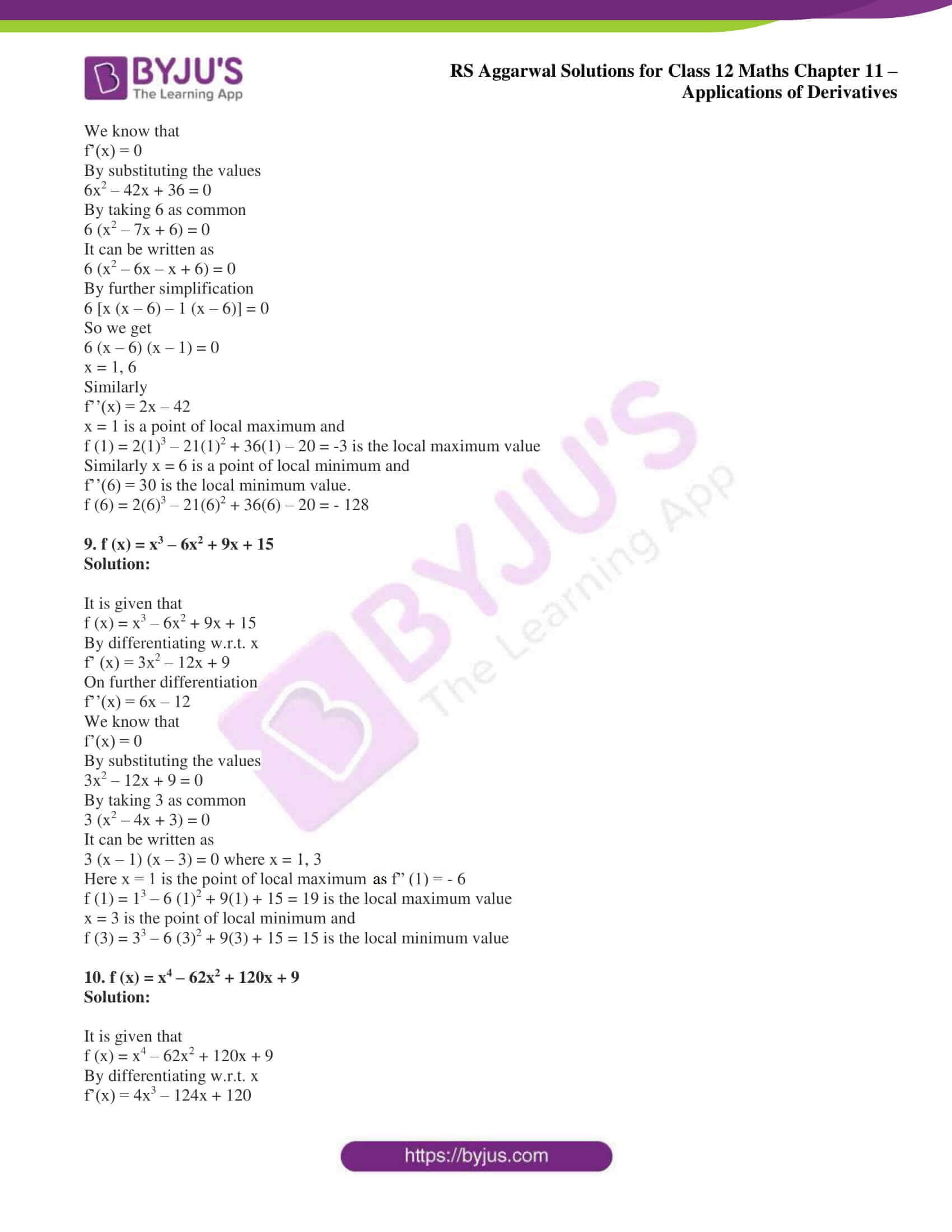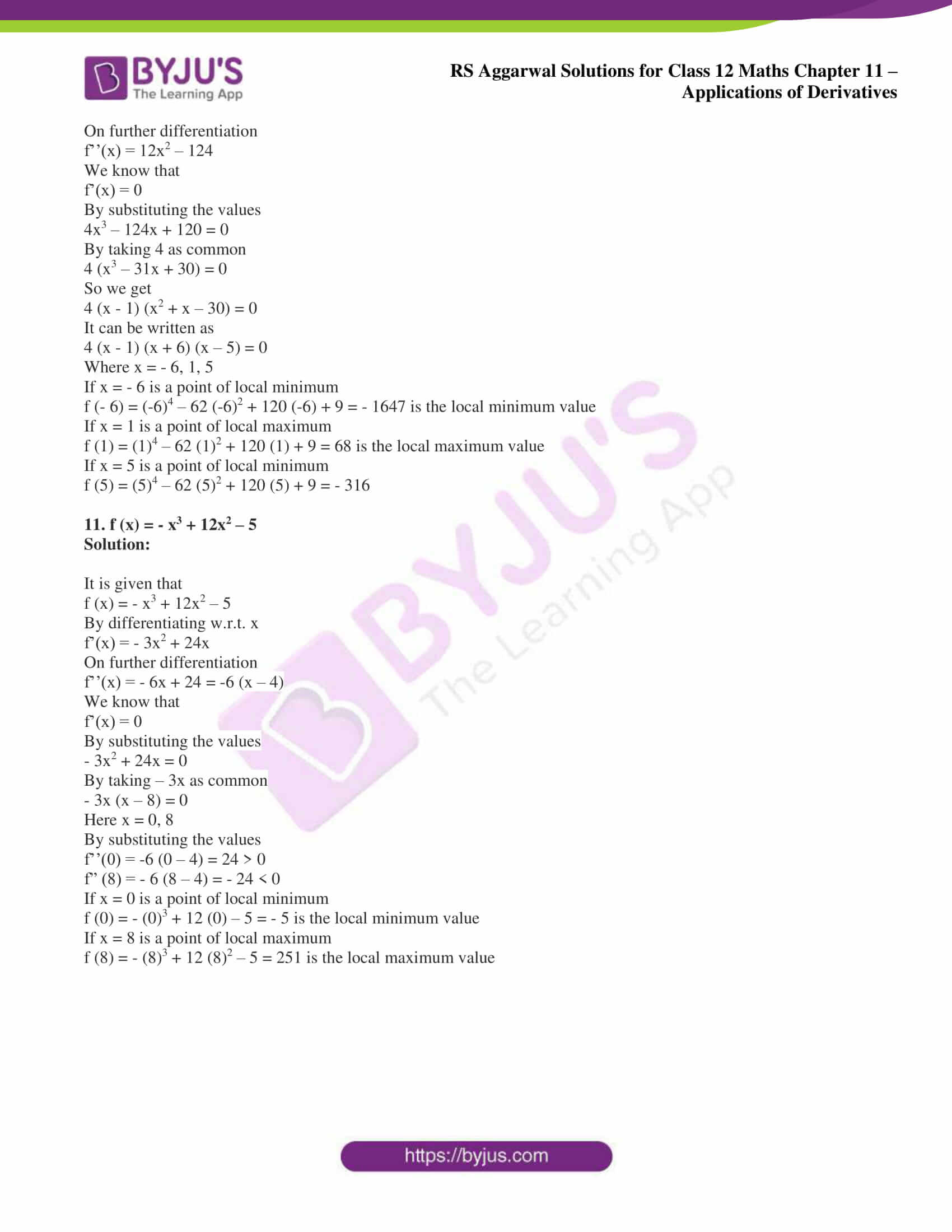# RS Aggarwal Solutions for Class 12 Maths Chapter 11: Applications of Derivatives Exercise 11E

Absolute maximum value, minimum value, local maximum value and minimum value of a function are the concepts discussed under the Exercise 11E of Chapter 11. The method of determining the maximum and minimum values without using derivatives are explained in brief under this exercise. In order to score good marks in the board exam, students can use RS Aggarwal Solutions Class 12 Maths Chapter 11 Applications of Derivatives Exercise 11E PDF from the links which are available below.

## RS Aggarwal Solutions for Class 12 Maths Chapter 11: Applications of Derivatives Exercise 11E Download PDF### Access other exercise solutions of Class 12 Maths Chapter 11: Applications of Derivatives

Exercise 11A Solutions

Exercise 11B Solutions

Exercise 11C Solutions

Exercise 11D Solutions

Exercise 11F Solutions

Exercise 11G Solutions

Exercise 11H Solutions

### Access RS Aggarwal Solutions for Class 12 Maths Chapter 11: Applications of Derivatives Exercise 11E

Find the maximum or minimum values, if any, without using derivatives, of the functions:

1. (5x – 1)2 + 4

Solution:

It is given that f (x) = (5x – 1)2 + 4 with minimum value 4 when, 5x – 1 = 0

Here the maximum value cannot be found i.e. when x increases even f (x) increases

Therefore, f (x) does not have a maximum value and has a minimum value of 4 at x = 1/5.

2. – (x – 3)2 + 9

Solution:

It is given that f (x) = – (x – 3)2 + 9

Here (x – 3)2 ≥ 0

We can write it as

– (x – 3)2 ≤ 0

By adding 9 on both sides

– (x – 3)2 + 9 ≤ 0 + 9

Where f (x) ≤ 9

So the maximum value of f (x) = 9 which occurs at x = 3 which is the point of absolute maxima and minimum value does not exist.

3. – |x + 4| + 6

Solution:

We can write it as

|x + 4| ≥ 0

By taking – ve sign

– |x + 4| ≤ 0

By adding 6 on both sides

– (x + 4) + 6 ≤ 0 + 6

Where f (x) ≤ 6

Here the maximum value of f (x) = 6 which occurs at x = – 4 which is the point of absolute maxima and minimum value of f (x) does not exists.

4. sin 2x + 5

Solution:

For all x

– 1 ≤ sin 2x ≤ 1

5 – 1 ≤ sin 2x + 5 ≤ 5 + 1

On further calculation

4 ≤ f (x) ≤ 6

Here the minimum value of f (x) = 4 and minimum value of f (x) = 6.

5. |sin 4x + 3|

Solution:

For all x

– 1 ≤ sin 4x ≤ 1

3 – 1 ≤ sin 4x + 3 ≤ 1 + 3

On further calculation

2 ≤ sin 4x + 3 ≤ 4

We know that

|2| ≤ |sin 4x + 3| ≤ |4|

2 ≤ f (x) ≤ 4

Here the minimum value of f (x) = 2 and maximum value of f (x) = 4.

Find the points of local maxima or local minima and the corresponding local maximum and minimum values of each of the following functions:

6. f (x) = (x – 3)4

Solution:

It is given that

f (x) = (x – 3)4

By differentiating w.r.t. x

f’(x) = 4 (x – 3)3

We know that

f’(x) = 0

By substituting the values

4 (x – 3)3 = 0

So we get

x – 3 = 0 where x = 3

f”(x) = 12 (x – 3)2 ≥ 0

x = 3 is point of local minima and local minimum value is f (3) = 0

7. f (x) = x2

Solution:

It is given that

f (x) = x2

By differentiating w.r.t. x

f’(x) = 2x and f”(x) = 2

We know that

f’(x) = 0

By substituting the values

2x = 0 where x = 0

f”(0) = 2 > 0

Hence, x = 0 is point of local minimum and f (0) = 0 is the local minimum value.

8. f (x) = 2x3 – 21x2 + 36x – 20

Solution:

It is given that

f (x) = 2x3 – 21x2 + 36x – 20

By differentiating w.r.t. x

f’(x) = 6x2 – 42x + 36

We know that

f’(x) = 0

By substituting the values

6x2 – 42x + 36 = 0

By taking 6 as common

6 (x2 – 7x + 6) = 0

It can be written as

6 (x2 – 6x – x + 6) = 0

By further simplification

6 [x (x – 6) – 1 (x – 6)] = 0

So we get

6 (x – 6) (x – 1) = 0

x = 1, 6

Similarly

f’’(x) = 2x – 42

x = 1 is a point of local maximum and

f (1) = 2(1)3 – 21(1)2 + 36(1) – 20 = -3 is the local maximum value

Similarly x = 6 is a point of local minimum and

f’’(6) = 30 is the local minimum value.

f (6) = 2(6)3 – 21(6)2 + 36(6) – 20 = – 128

9. f (x) = x3 – 6x2 + 9x + 15

Solution:

It is given that

f (x) = x3 – 6x2 + 9x + 15

By differentiating w.r.t. x

f’ (x) = 3x2 – 12x + 9

On further differentiation

f’’(x) = 6x – 12

We know that

f’(x) = 0

By substituting the values

3x2 – 12x + 9 = 0

By taking 3 as common

3 (x2 – 4x + 3) = 0

It can be written as

3 (x – 1) (x – 3) = 0 where x = 1, 3

Here x = 1 is the point of local maximum as f” (1) = – 6

f (1) = 13 – 6 (1)2 + 9(1) + 15 = 19 is the local maximum value

x = 3 is the point of local minimum and

f (3) = 33 – 6 (3)2 + 9(3) + 15 = 15 is the local minimum value

10. f (x) = x4 – 62x2 + 120x + 9

Solution:

It is given that

f (x) = x4 – 62x2 + 120x + 9

By differentiating w.r.t. x

f’(x) = 4x3 – 124x + 120

On further differentiation

f’’(x) = 12x2 – 124

We know that

f’(x) = 0

By substituting the values

4x3 – 124x + 120 = 0

By taking 4 as common

4 (x3 – 31x + 30) = 0

So we get

4 (x – 1) (x2 + x – 30) = 0

It can be written as

4 (x – 1) (x + 6) (x – 5) = 0

Where x = – 6, 1, 5

If x = – 6 is a point of local minimum

f (- 6) = (-6)4 – 62 (-6)2 + 120 (-6) + 9 = – 1647 is the local minimum value

If x = 1 is a point of local maximum

f (1) = (1)4 – 62 (1)2 + 120 (1) + 9 = 68 is the local maximum value

If x = 5 is a point of local minimum

f (5) = (5)4 – 62 (5)2 + 120 (5) + 9 = – 316

11. f (x) = – x3 + 12x2 – 5

Solution:

It is given that

f (x) = – x3 + 12x2 – 5

By differentiating w.r.t. x

f’(x) = – 3x2 + 24x

On further differentiation

f’’(x) = – 6x + 24 = -6 (x – 4)

We know that

f’(x) = 0

By substituting the values

– 3x2 + 24x = 0

By taking – 3x as common

– 3x (x – 8) = 0

Here x = 0, 8

By substituting the values

f’’(0) = -6 (0 – 4) = 24 > 0

f” (8) = – 6 (8 – 4) = – 24 < 0

If x = 0 is a point of local minimum

f (0) = – (0)3 + 12 (0) – 5 = – 5 is the local minimum value

If x = 8 is a point of local maximum

f (8) = – (8)3 + 12 (8)2 – 5 = 251 is the local maximum value